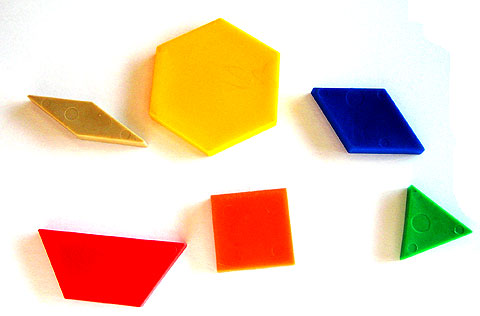Home > Patterns > Big ideas > Patterns in shapes > The language of shapes

# The language of shapes

Every early mathematics classroom should have a plentiful supply of pattern blocks.A set of pattern blocks.

Blocks made of thick felt are quieter than blocks made of wood or plastic.

Pattern blocks may be used to introduce students to some basic geometrical concepts that arise from the patterns within the shapes. For example, you could give each student a set of six different blocks and ask them to discuss the following questions.

• Look at each block in turn. What is regular about it?
• Choose any two blocks. What is the same about them? How are they different?
• Sort the pattern blocks into groups. What is the same about all the blocks in each group?

Initially, it is best to use everyday language, such as:

 length edges corners triangle square straight same.

Geometrical language can then be gradually introduced, such as:

 side vertex angle congruent perpendicular parallel symmetrical acute obtuse regular rhombus trapezium hexagon.

Notice that the term 'regular polygon' has a special meaning in geometry; it is a polygon with all sides and angles equal. In that sense, only the triangle, square and hexagon are regular polygons. Nevertheless, the other figures do have some regularity.

Pattern blocks may also be used to make patterns where the same basic geometrical ideas also occur.